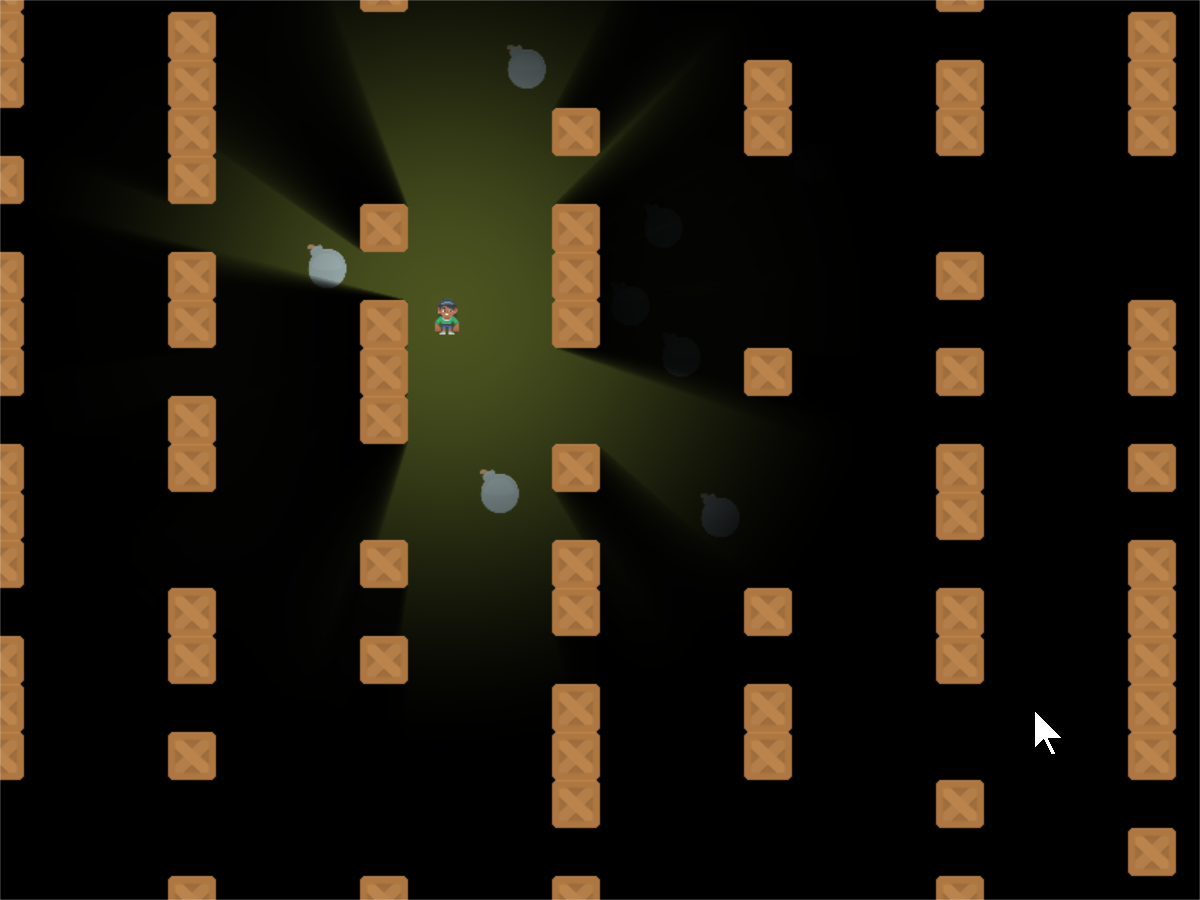# Step 6 GLSL#step_06.glsl#
 ``` 1 2 3 4 5 6 7 8 9 10 11 12 13 14 15 16 17 18 19 20 21 22 23 24 25 26 27 28 29 30 31 32 33 34 35 36 37 38 39 40 41 42 43 44 45 46 47 48 49 50 51 52 53 54``` ```#define N 500 // x, y position of the light uniform vec2 lightPosition; // Size of light in pixels uniform float lightSize; float terrain(vec2 samplePoint) { float samplePointAlpha = texture(iChannel0, samplePoint).a; float sampleStepped = step(0.1, samplePointAlpha); float returnValue = 1.0 - sampleStepped; // Soften the shadows. Comment out for hard shadows. // The closer the first number is to 1.0, the softer the shadows. returnValue = mix(0.98, 1.0, returnValue); return returnValue; } void mainImage( out vec4 fragColor, in vec2 fragCoord ) { // Distance in pixels to the light float distanceToLight = length(lightPosition - fragCoord); // Normalize the fragment coordinate from (0.0, 0.0) to (1.0, 1.0) vec2 normalizedFragCoord = fragCoord/iResolution.xy; vec2 normalizedLightCoord = lightPosition.xy/iResolution.xy; // Start our mixing variable at 1.0 float lightAmount = 1.0; for(float i = 0.0; i < N; i++) { // A 0.0 - 1.0 ratio between where our current pixel is, and where the light is float t = i / N; // Grab a coordinate between where we are and the light vec2 samplePoint = mix(normalizedFragCoord, normalizedLightCoord, t); // Is there something there? If so, we'll assume we are in shadow float shadowAmount = terrain(samplePoint); // Multiply the light amount. // (Multiply in case we want to upgrade to soft shadows) lightAmount *= shadowAmount; } // Find out how much light we have based on the distance to our light lightAmount *= 1.0 - smoothstep(0.0, lightSize, distanceToLight); // We'll alternate our display between black and whatever is in channel 1 vec4 blackColor = vec4(0.0, 0.0, 0.0, 1.0); // Our fragment color will be somewhere between black and channel 1 // dependent on the value of b. fragColor = mix(blackColor, texture(iChannel1, normalizedFragCoord), lightAmount); } ```